## Create Line Plot with Markers

Adding markers to a line plot can be a useful way to distinguish multiple lines or to highlight particular data points. Add markers in one of these ways:

• Include a marker symbol in the line-specification input argument, such as `plot(x,y,'-s')`.

• Specify the `Marker` property as a name-value pair, such as `plot(x,y,'Marker','s')`.

For a list of marker options, see Supported Marker Symbols.

### Add Markers to Line Plot

Create a line plot. Display a marker at each data point by including the line-specification input argument when calling the `plot` function. For example, use `'-o'` for a solid line with circle markers.

```x = linspace(0,10,100); y = exp(x/10).*sin(4*x); plot(x,y,'-o')```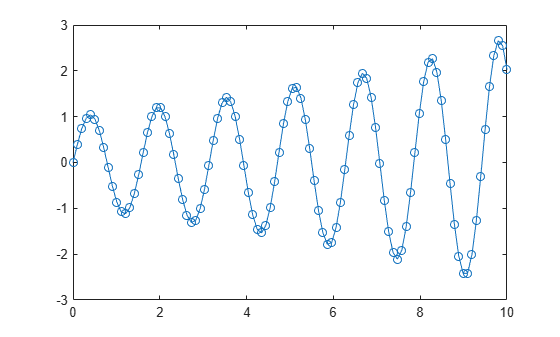If you specify a marker symbol and do not specify a line style, then `plot` displays only the markers with no line connecting them.

`plot(x,y,'o')`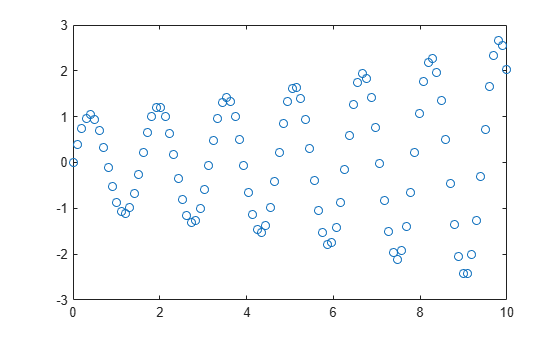Alternatively, you can add markers to a line by setting the `Marker` property as a name-value pair. For example, `plot(x,y,'Marker','o')` plots a line with circle markers.

### Specify Marker Size and Color

Create a line plot with markers. Customize the markers by setting these properties using name-value pair arguments with the `plot` function:

• `MarkerSize` - Marker size, which is specified as a positive value.

• `MarkerEdgeColor` - Marker outline color, which is specified as a color name or an RGB triplet.

• `MarkerFaceColor` - Marker interior color, which is specified as a color name or an RGB triplet.

Specify the colors using either a character vector of a color name, such as `'red'`, or an RGB triplet, such as `[0.4 0.6 0.7]`. An RGB triplet is a three-element row vector whose elements specify the intensities of the red, green, and blue components of the color. The intensities must be in the range [0,1].

```x = linspace(0,10,50); y = sin(x); plot(x,y,'-s','MarkerSize',10,... 'MarkerEdgeColor','red',... 'MarkerFaceColor',[1 .6 .6])```### Control Placement of Markers Along Line

Create a line plot with 1,000 data points, add asterisks markers, and control the marker positions using the `MarkerIndices` property. Set the property to the indices of the data points where you want to display markers. Display a marker every tenth data point, starting with the first data point.

```x = linspace(0,10,1000); y = exp(x/10).*sin(4*x); plot(x,y,'-*','MarkerIndices',1:10:length(y))```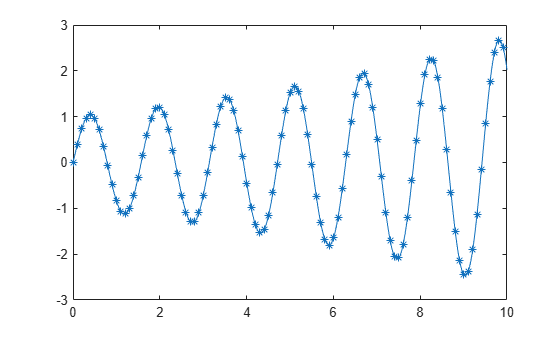### Display Markers at Maximum and Minimum Data Points

Create a vector of random data and find the index of the minimum and maximum values. Then, create a line plot of the data. Display red markers at the minimum and maximum data values by setting the `MarkerIndices` property to a vector of the index values.

```x = 1:100; y = rand(100,1); idxmin = find(y == max(y)); idxmax = find(y == min(y)); plot(x,y,'-p','MarkerIndices',[idxmin idxmax],... 'MarkerFaceColor','red',... 'MarkerSize',15)```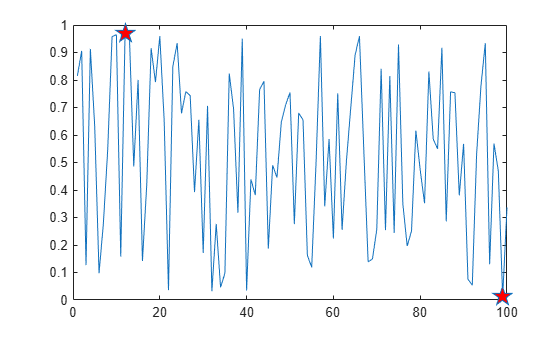### Revert to Default Marker Locations

Modify the marker locations, then revert back to the default locations.

Create a line plot and display large, square markers every five data points. Assign the chart line object to the variable `p` so that you can access its properties after it is created.

```x = linspace(0,10,25); y = x.^2; p = plot(x,y,'-s'); p.MarkerSize = 10; p.MarkerIndices = 1:5:length(y);```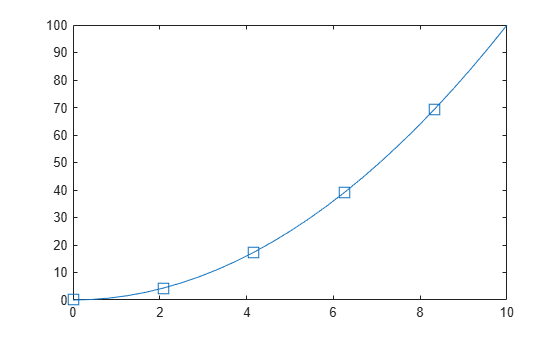Reset the `MarkerIndices` property to the default value, which is a vector of all index values from 1 to the number of data points.

`p.MarkerIndices = 1:length(y);`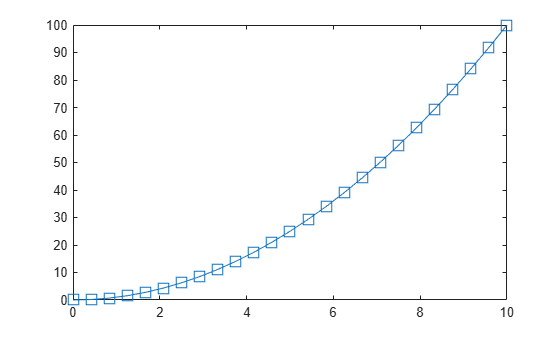### Supported Marker Symbols

ValueDescription
`'o'`Circle
`'+'`Plus sign
`'*'`Asterisk
`'.'`Point
`'x'`Cross
`'_'`Horizontal line
`'|'`Vertical line
`'square'` or `'s'`Square
`'diamond'` or `'d'`Diamond
`'^'`Upward-pointing triangle
`'v'`Downward-pointing triangle
`'>'`Right-pointing triangle
`'<'`Left-pointing triangle
`'pentagram'` or `'p'`Five-pointed star (pentagram)
`'hexagram'` or `'h'`Six-pointed star (hexagram)
`'none'`No markers

The line-specification input argument does not support marker options that are more than one character. Use the one character alternative or set the `Marker` property instead.